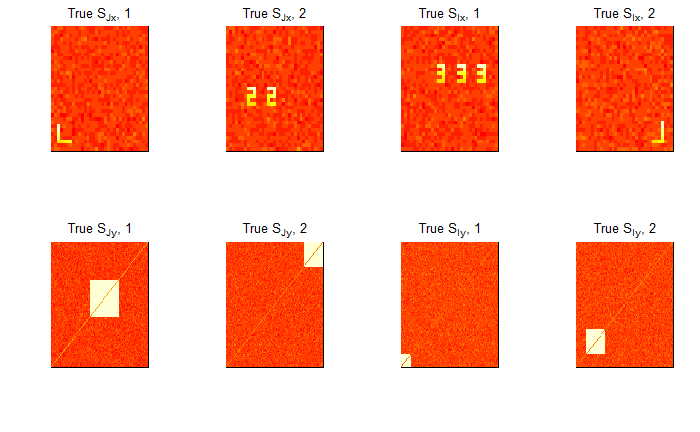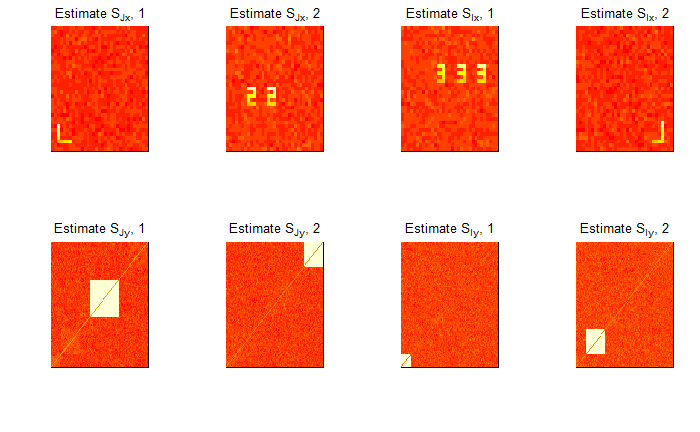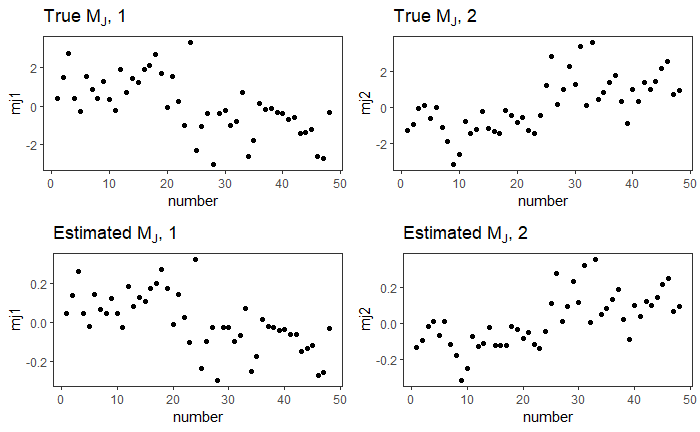# singR

singR package is built on SING method https://github.com/thebrisklab/SING. SING is used to extract joint and individual non-gaussian components from different datasets. This is a tutorial example supporting the paper Simultaneous Non-Gaussian Component Analysis (SING) for Data Integration in Neuroimaging Benjamin Risk, Irina Gaynanova https://arxiv.org/abs/2005.00597v1

## Installation

You can install singR from github with:

library(devtools)
install_github("thebrisklab/singR")

If you want to install it on Mac OS, the installation tips is here:

1.Make sure all the R packages used in singR are updated to the recommended version.

2.Try to install singR, if there is an error saying:

ld: warning: directory not found for option '-L/opt/R/arm64/gfortran/lib/gcc/aarch64-apple-darwin20.2.0/11.0.0'
clang: error: linker command failed with exit code 1 (use -v to see invocation)

Then go to: /Library/Frameworks/R.framework/Resources/etc/Makeconf Change FLIBS from

FLIBS =  -L/opt/R/arm64/gfortran/lib/gcc/aarch64-apple-darwin20.2.0/11.0.0 -L/opt/R/arm64/gfortran/lib -lgfortran -lemutls_w -lm

to

FLIBS =  -L/usr/local/gfortran/lib/gcc/aarch64-apple-darwin20.2.0/11.0.0 -L/usr/local/gfortran/lib -lgfortran -lm

3.Download the .tar.xz file from https://github.com/fxcoudert/gfortran-for-macOS/releases/tag/11-arm-alpha2 using the browser, unpack it under /usr/local so that the “gfortran” folder is there.

4.Install singR again, it should be installed successfully.

## Quick Start Guide

To illustrate the use of , we provide an example .

The tutorial dataset exampledata are included in the package. We generate the SING model in @ref(eq:two) as follows. We generate joint subject scores $$M_{J}=[m_{J1},m_{J2}]\in \mathbb{R}^{n\times2}$$ with $$m_{J1}\sim N(\mu_{1},I_{n}),m_{J2}\sim N(\mu_{2},I_{n})$$, $$\mu_{1}=(1_{24}^{\top},-1_{24}^{\top})^{\top}$$ and $$\mu_{2}=(-1_{24}^{\top},1_{24}^{\top})^{\top}$$. We set $$D_{x}=I$$ and $$D_{y}=diag(-5,2)$$ to have differences in both sign and scale between the two datasets. We generate $$M_{Ix}$$ and $$M_{Iy}$$ similar to $$M_{J}$$ using iid unit variance Gaussian entries with means equal to $$\mu_{3y}=(-1_{6}^{\top},1_{6}^{\top},-1_{6}^{\top},1_{6}^{\top},-1_{6}^{\top},1_{6}^{\top}-1_{6}^{\top},-1_{6}^{\top})^{\top}$$, $$\mu_{4y}=(1_{24}^{\top},-1_{24}^{\top})^{\top}$$, $$\mu_{3x}=(-1_{12}^{\top},1_{12}^{\top},-1_{12}^{\top},1_{12}^{\top})^{\top}$$, $$\mu_{4x}=(1_{12}^{\top},-1_{12}^{\top},1_{12}^{\top},-1_{12}^{\top})^{\top}$$. These means result in various degrees of correlation between the columns of the mixing matrices. For the Gaussian noise, we generate $$M_{Nx}$$, $$M_{Ny}$$, $$N_{x}$$ and $$N_{y}$$ using iid standard Gaussian mean zero entries.

library(singR)
data(exampledata)
data <- exampledata

lgrid = 33
par(mfrow = c(2, 4))
# Components for X
image(matrix(data$sjX[1, ], lgrid, lgrid), col = heat.colors(12), xaxt = "n", yaxt = "n", main = expression("True S"["Jx"] * ", 1")) image(matrix(data$sjX[2, ], lgrid, lgrid), col = heat.colors(12), xaxt = "n",
yaxt = "n", main = expression("True S"["Jx"] * ", 2"))
image(matrix(data$siX[1, ], lgrid, lgrid), col = heat.colors(12), xaxt = "n", yaxt = "n", main = expression("True S"["Ix"] * ", 1")) image(matrix(data$siX[2, ], lgrid, lgrid), col = heat.colors(12), xaxt = "n",
yaxt = "n", main = expression("True S"["Ix"] * ", 2"))

# Components for Y
image(vec2net(data$sjY[1, ]), col = heat.colors(12), xaxt = "n", yaxt = "n", main = expression("True S"["Jy"] * ", 1")) image(vec2net(data$sjY[2, ]), col = heat.colors(12), xaxt = "n", yaxt = "n",
main = expression("True S"["Jy"] * ", 2"))
image(vec2net(data$siY[1, ]), col = heat.colors(12), xaxt = "n", yaxt = "n", main = expression("True S"["Iy"] * ", 1")) image(vec2net(data$siY[2, ]), col = heat.colors(12), xaxt = "n", yaxt = "n",
main = expression("True S"["Iy"] * ", 2"))Function singR performs all steps in the SING pipeline as a single function

We first illustrate the use of the wrapper function singR using the default settings. We will describe optional arguments in more detail in example 2.

example1 = singR(dX = data$dX, dY = data$dY, individual = T)

Details of the SING pipeline

We next explain each of the steps involved in SING estimation. Using these individual functions in place of the high-level singR function allows additional fine-tuning and can be helpful for large datasets.

Estimate the number of non-Gaussian components in datasets dX and dY using FOBIasymp from :

n.comp.X = NG_number(data$dX) n.comp.Y = NG_number(data$dY)

Apply lngca separately to each dataset using the JB statistic as the measure of non-Gaussianity:

# JB on X
estX_JB = lngca(xData = data$dX, n.comp = n.comp.X, whiten = "sqrtprec", restarts.pbyd = 20, distribution = "JB") Uxfull <- estX_JB$U
Mx_JB = est.M.ols(sData = estX_JB$S, xData = data$dX)

# JB on Y
estY_JB = lngca(xData = data$dY, n.comp = n.comp.Y, whiten = "sqrtprec", restarts.pbyd = 20, distribution = "JB") Uyfull <- estY_JB$U
My_JB = est.M.ols(sData = estY_JB$S, xData = data$dY)

Use greedymatch to reorder $$\widehat{U}_{x}$$ and $$\widehat{U}_{y}$$ by descending matched correlations and use permTestJointRank to estimate the number of joint components:

matchMxMy = greedymatch(scale(Mx_JB, scale = F), scale(My_JB, scale = F),
Ux = Uxfull, Uy = Uyfull)
permJoint <- permTestJointRank(matchMxMy$Mx, matchMxMy$My)
joint_rank = permJoint$rj For preparing input to curvilinear_c, manually prewhiten dX and dY to get $$\widehat{L}_{x}^{-1}$$ and $$\widehat{L}_{y}^{-1}$$: # Center X and Y dX = data$dX
dY = data$dY n = nrow(dX) pX = ncol(dX) pY = ncol(dY) dXcentered <- dX - matrix(rowMeans(dX), n, pX, byrow = F) dYcentered <- dY - matrix(rowMeans(dY), n, pY, byrow = F) # For X Scale rowwise est.sigmaXA = tcrossprod(dXcentered)/(pX - 1) whitenerXA = est.sigmaXA %^% (-0.5) xDataA = whitenerXA %*% dXcentered invLx = est.sigmaXA %^% (0.5) # For Y Scale rowwise est.sigmaYA = tcrossprod(dYcentered)/(pY - 1) whitenerYA = est.sigmaYA %^% (-0.5) yDataA = whitenerYA %*% dYcentered invLy = est.sigmaYA %^% (0.5) Obtain a reasonable value for the penalty $$\rho$$ by calculating the JB statistics for all the joint components: # Calculate the Sx and Sy. Sx = matchMxMy$Ux[1:joint_rank, ] %*% xDataA
Sy = matchMxMy$Uy[1:joint_rank, ] %*% yDataA JBall = calculateJB(Sx) + calculateJB(Sy) # Penalty used in curvilinear algorithm: rho = JBall/10 Estimate $$\widehat{U}_{x}$$ and $$\widehat{U}_{y}$$ with curvilinear_c: # alpha=0.8 corresponds to JB weighting of skewness and kurtosis (can # customize to use different weighting): alpha = 0.8 # tolerance: tol = 1e-10 out <- curvilinear_c(invLx = invLx, invLy = invLy, xData = xDataA, yData = yDataA, Ux = matchMxMy$Ux, Uy = matchMxMy$Uy, rho = rho, tol = tol, alpha = alpha, maxiter = 1500, rj = joint_rank) Obtain the final result: # Estimate Sx and Sy and true S matrix Sjx = out$Ux[1:joint_rank, ] %*% xDataA
Six = out$Ux[(joint_rank + 1):n.comp.X, ] %*% xDataA Sjy = out$Uy[1:joint_rank, ] %*% yDataA
Siy = out$Uy[(joint_rank + 1):n.comp.Y, ] %*% yDataA # Estimate Mj and true Mj Mxjoint = tcrossprod(invLx, out$Ux[1:joint_rank, ])
Mxindiv = tcrossprod(invLx, out$Ux[(joint_rank + 1):n.comp.X, ]) Myjoint = tcrossprod(invLy, out$Uy[1:joint_rank, ])
Myindiv = tcrossprod(invLy, out$Uy[(joint_rank + 1):n.comp.Y, ]) # signchange to keep all the S and M skewness positive Sjx_sign = signchange(Sjx, Mxjoint) Sjy_sign = signchange(Sjy, Myjoint) Six_sign = signchange(Six, Mxindiv) Siy_sign = signchange(Siy, Myindiv) Sjx = Sjx_sign$S
Sjy = Sjy_sign$S Six = Six_sign$S
Siy = Siy_sign$S Mxjoint = Sjx_sign$M
Myjoint = Sjy_sign$M Mxindiv = Six_sign$M
Myindiv = Siy_sign$M est.Mj = aveM(Mxjoint, Myjoint) trueMj <- data.frame(mj1 = data$mj[, 1], mj2 = data\$mj[, 2], number = 1:48)
SINGMj <- data.frame(mj1 = est.Mj[, 1], mj2 = est.Mj[, 2], number = 1:48)

Plot $$\widehat{S}_{Jx}$$, $$\widehat{S}_{Jy}$$, $$\widehat{S}_{Ix}$$, and $$\widehat{S}_{Iy}$$ in figure @ref(fig:estiexample1).Plot $$\widehat{M}_J$$ in figure @ref(fig:mjex1).

library(tidyverse)
library(ggpubr)

t1 <- ggplot(data = trueMj) + geom_point(mapping = aes(y = mj1, x = number)) +
ggtitle(expression("True M"["J"] * ", 1")) + theme_bw() + theme(panel.grid = element_blank())

t2 <- ggplot(data = trueMj) + geom_point(mapping = aes(y = mj2, x = number)) +
ggtitle(expression("True M"["J"] * ", 2")) + theme_bw() + theme(panel.grid = element_blank())

# SING mj

S1 <- ggplot(data = SINGMj) + geom_point(mapping = aes(y = mj1, x = number)) +
ggtitle(expression("Estimated M"["J"] * ", 1")) + theme_bw() + theme(panel.grid = element_blank())

S2 <- ggplot(data = SINGMj) + geom_point(mapping = aes(y = mj2, x = number)) +
ggtitle(expression("Estimated M"["J"] * ", 2")) + theme_bw() + theme(panel.grid = element_blank())

ggarrange(t1, t2, S1, S2, ncol = 2, nrow = 2)Estimated joint subject scores in example 1.

## Acknowledgments

Research reported in this publication was supported by the National Institute of Mental Health of the National Institutes of Health under award number R01MH129855 to BBR. The research was also supported by the Division of Mathematical Sciences of the National Science Foundation under award number DMS-2044823 to IG. The content is solely the responsibility of the authors and does not necessarily represent the official views of the National Institutes of Health and National Science Foundation.